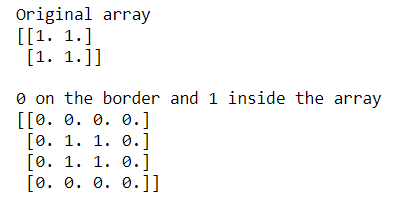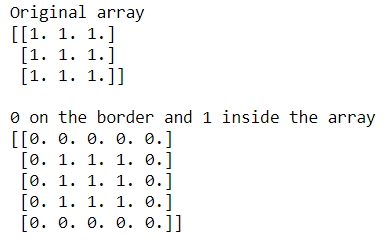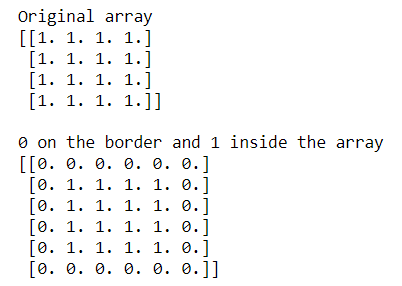# How to add a border around a NumPy array?

Sometimes we need to add a border around a NumPy matrix. Numpy provides a function known as ‘numpy.pad()’ to construct the border. The below examples show how to construct a border of ‘0’ around the identity matrix.

Syntax :

```numpy.pad(array, pad_width, mode='constant', **kwargs)
```

Example 1: Construct a border of 0s around 2D identity matrix

## Python3

 `# importing Numpy package ` `import` `numpy as np ` ` `  `# Creating a 2X2 Numpy matrix ` `array ``=` `np.ones((``2``, ``2``)) ` ` `  `print``(``"Original array"``) ` `print``(array) ` ` `  `print``(``"\n0 on the border and 1 inside the array"``) ` ` `  `# constructing border of 0 around 2D identity matrix ` `# using np.pad() ` `array ``=` `np.pad(array, pad_width``=``1``, mode``=``'constant'``, ` `               ``constant_values``=``0``) ` ` `  `print``(array) `

Output:In the above examples, we construct a border of 0s around the 2-D NumPy matrix.

Example 2: Construct a border of 0s around 3D identity matrix

## Python3

 `# importing Numpy package ` `import` `numpy as np ` ` `  `# Creating a 3X3 Numpy matrix ` `array ``=` `np.ones((``3``, ``3``)) ` ` `  `print``(``"Original array"``) ` `print``(array) ` ` `  `print``(``"\n0 on the border and 1 inside the array"``) ` ` `  `# constructing border of 0 around 3D identity matrix ` `# using np.pad() ` `array ``=` `np.pad(array, pad_width``=``1``, mode``=``'constant'``, ` `               ``constant_values``=``0``) ` ` `  `print``(array) `

Output:In the above examples, we construct a border of 0s around the 3-D NumPy matrix.

Example 3: Construct a border of 0s around 4D identity matrix

## Python3

 `# importing Numpy package ` `import` `numpy as np ` ` `  `# Creating a 4X4 Numpy matrix ` `array ``=` `np.ones((``4``, ``4``)) ` ` `  `print``(``"Original array"``) ` `print``(array) ` ` `  `print``(``"\n0 on the border and 1 inside the array"``) ` ` `  `# constructing border of 0 around 4D identity matrix ` `# using np.pad() ` `array ``=` `np.pad(array, pad_width``=``1``, mode``=``'constant'``, ` `               ``constant_values``=``0``) ` ` `  `print``(array) `

Output:In the above examples, we construct a border of 0s around the 4-D NumPy matrix.

Whether you're preparing for your first job interview or aiming to upskill in this ever-evolving tech landscape, GeeksforGeeks Courses are your key to success. We provide top-quality content at affordable prices, all geared towards accelerating your growth in a time-bound manner. Join the millions we've already empowered, and we're here to do the same for you. Don't miss out - check it out now!

Previous
Next